# Bessel Functions

The following article is from The Great Soviet Encyclopedia (1979). It might be outdated or ideologically biased.

## Bessel Functions

cylinder functions of the first kind; they occur in the consideration of physical processes (heat conduction, diffusion, oscillations, and so on) in areas with circular and cylindrical symmetry. They are solutions of the Bessel equation.

The Bessel function Jp of the order (index) p,- ∞ < p < ∞, is represented by the series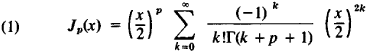which converges for all x. Its graph for x > 0 has the form of a damped oscillation; Jp(x) has an infinite number of zeros. The behavior of Jp (x) for small | x | is given by the first term of the series (1); for large x > 0, the following asymptotic representation holds: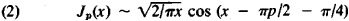Here the oscillatory character of the function is clearly shown. The Bessel functions of the “half-integer” order p = n + ½ are expressed in terms of elementary functions—in particular,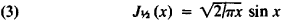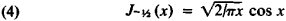The Bessel functions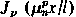, where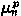are the positive zeros of Jp(x) and where p > - ½, form an orthogonal system with weight × in the interval (0, l).

The function J0 was first considered by D. Bernoulli in a paper devoted to the oscillation of heavy chains (1732). L. Euler, considering the problem of the oscillations of a circular membrane (1738), arrived at the Bessel equation with whole values p = n and found an expression for Jn(x) in the form of a series of the powers of x. In later works he extended this expression to the case of arbitrary ρ values. F. Bessel investigated the functions Jp (x) in connection with the study of the motion of the planets around the sun (1824). He compiled the first tables for J0 (x), J1 (x), and J2 (x).

### REFERENCES

Watson, G. N. Teoriia besselevykh funktsii, parts 1–2. Moscow, 1949. (Translated from English.)
Lebedev, N. N. Spetsial’nye funktsii i ikh prilozheniia, 2nd ed. Moscow-Leningrad, 1963.
Bateman, H., and A. Erdély. Vysshie transtsendentnye funktsii: Funktsii Besselia, funktsii parabolicheskogo tsilindra, orto-gonal’nye mnogochleny. Moscow, 1966. (Translated from English.) P. I. Lizorkin
The Great Soviet Encyclopedia, 3rd Edition (1970-1979). © 2010 The Gale Group, Inc. All rights reserved.
References in periodicals archive ?
One of the formulas is given in [5, Theorem 1.1] and expressed as an infinite sum of which each summand consists of the modified Bessel functions, the Gegenbauer polynomials and the densities [p.sub.r.sup(0)] (.;x).
where [J.sub.0] and [J.sub.1] are Bessel functions of the first kind and of order zero and one respectively.
There Gautschi developed a number of computer algorithms for evaluating special functions, for example, for the gamma and incomplete gamma functions, and Bessel functions of the first kind.
where x = r cos([theta]) and y = r sin([theta]), [J.sub.[mu]+n] are Bessel functions of first kind, [C.sup.[mu].sub.n] are Gegenbauer polynomials, and [GAMMA](2[mu]) = [2.sup.[mu]-1] ([mu]-1)!.
A substantial number of applications arise from the separation of variables in the Laplace operator in cylindrical and spherical coordinates that leads, respectively, to the cylindrical and spherical Bessel functions. The cases of specific interest include two types of cylindrical waves: (i) sound waves as compressible perturbations of a uniform flow; (ii) vortical waves as incompressible perturbations of a uniform flow with superimposed rigid body rotation.
Mostafa  and Porwal and Dixit  obtain certain conditions for hypergeometric functions and generalized Bessel functions, respectively, for these classes.
Further, the generalized Bessel function defined in (1) possesses the lead that a number of Bessel functions, trigonometric functions, and hyperbolic functions happen to be the particular cases of this function.
Geometric properties of special functions such as hypergeometric functions, Bessel functions, Struve functions, Mittag-Leffler functions, Wright functions, and some other related functions are an ongoing part of research in geometric function theory.
Based on the above analysis, one can find that the radiated fields are mainly characterized by the Bessel functions ([J.sub.l](*) and [J'.sub.l](*)) and the phase factor [e.sup.jl([phi]+[pi]/2)].
here [mathematical expression not reproducible] are the modified Bessel functions of the first and second kind, respectively.
The author covers Bessel and associated functions, generating functions of Bessel and associated Bessel functions, convergence of series in Bessel functions, and a wide variety of related subjects.
In nonstationary signal processing, another function basis widely used in signal decomposition is the Bessel function. The Bessel functions form the orthogonal basis and decay over the time, so that the signals which do not overlap in both the time and the frequency domain, including single frequency signals and linear frequency modulated (LFM) signals, can be represented well using the Fourier-Bessel transform (FBT) or the Fourier-Bessel (FB) series expansion [15-18].

Site: Follow: Share:
Open / Close